Formal DefinitionsNext: Example Queries Up: The Domain Relational Previous: The Domain Relational

## Formal Definitions

1. An expression is of the form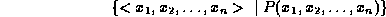where therepresent domain variables, and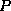is a formula.

2. An atom in the domain relational calculus is of the following forms
•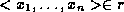whereis a relation onattributes, and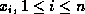, are domain variables or constants.
•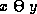, whereandare domain variables, and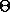is a comparison operator.

•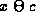, where c is a constant.
3. Formulae are built up from atoms using the following rules:
• An atom is a formula.
• Ifis a formula, then so are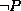and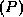.

• If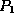and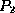are formulae, then so are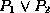,and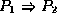.
• If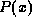is a formula where x is a domain variable, then so are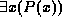and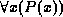.

Page created and maintained by Osmar R. Zaï ane
Last Update: Wed Sep 20 15:45:57 PDT 1995